# Simplification And Approximation-MCQ Test

## 10 Questions MCQ Test UPSC Prelims Paper 2 CSAT - Quant, Verbal & Decision Making | Simplification And Approximation-MCQ Test

Description
Attempt Simplification And Approximation-MCQ Test | 10 questions in 20 minutes | Mock test for Banking Exams preparation | Free important questions MCQ to study UPSC Prelims Paper 2 CSAT - Quant, Verbal & Decision Making for Banking Exams Exam | Download free PDF with solutions
QUESTION: 1

### In a regular week, there are 5 working days and for each day, the working hours are 8. A man gets Rs. 2.40 per hour for regular work and Rs. 3.20 per hours for overtime. If he earns Rs. 432 in 4 weeks, then how many hours does he work for ?

Solution:

Suppose the man works overtime for x hours.

Now, working hours in 4 weeks = (5 x 8 x 4) = 160.

∴ 160 x 2.40 + x x 3.20 = 432

⇒ 3.20x = 432 - 384 = 48

⇒ x = 15.

Hence, total hours of work = (160 + 15) = 175.

QUESTION: 2

### Free notebooks were distributed equally among children of a class. The number of notebooks each child got was one-eighth of the number of children. Had the number of children been half, each child would have got 16 notebooks. Total how many notebooks were distributed ?

Solution:

Let total number of children be x.

Then, x x(1/8)x = (x/2)x 16   ⇔  x = 64

Number of notebooks =(1/8)x2 =(1/8x 64 x 64)= 512

QUESTION: 3

### A man has some hens and cows. If the number of heads be 48 and the number of feet equals 140, then the number of hens will be:

Solution:

Let the number of hens be x and the number of cows be y.

Then, x + y = 48 .... (i)

and 2x + 4y = 140   ⇒   x + 2y = 70 .... (ii)

Solving (i) and (ii) we get: x = 26, y = 22.

∴ The required answer = 26.

QUESTION: 4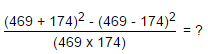Solution: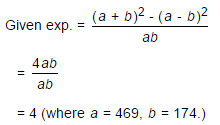QUESTION: 5

David gets on the elevator at the 11th floor of a building and rides up at the rate of 57 floors per minute. At the same time, Albert gets on an elevator at the 51st floor of the same building and rides down at the rate of 63 floors per minute. If they continue travelling at these rates, then at which floor will their paths cross ?

Solution:

Suppose their paths cross after x minutes.

Then, 11 + 57x = 51 - 63x    ⇔    120x = 40

x =1/3

Number of floors covered by David in (1/3) min. =((1/3)x 57)= 19

So, their paths cross at (11+19) i.e., 30th floor.

QUESTION: 6

A sum of Rs. 1360 has been divided among A, B and C such that A gets (2/3) of what B gets and B gets (1/4) of what C gets. B's share is:

Solution:

Let C's share = Rs. x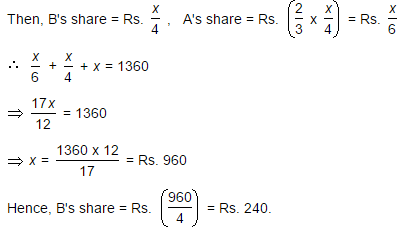QUESTION: 7

One-third of Rahul's savings in National Savings Certificate is equal to one-half of his savings in Public Provident Fund. If he has Rs. 1,50,000 as total savings, how much has he saved in Public Provident Fund ?

Solution:

Let savings in N.S.C and P.P.F. be Rs. x and Rs. (150000 - x) respectively. Then,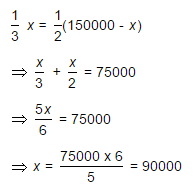Savings in Public Provident Fund = Rs. (150000 - 90000) = Rs. 60000

QUESTION: 8

A fires 5 shots to B's 3 but A kills only once in 3 shots while B kills once in 2 shots. When B has missed 27 times, A has killed:

Solution: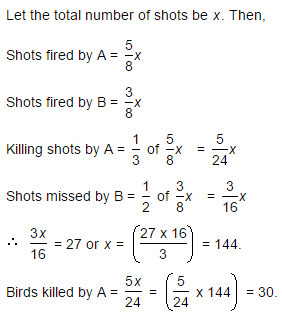QUESTION: 9

Eight people are planning to share equally the cost of a rental car. If one person withdraws from the arrangement and the others share equally the entire cost of the car, then the share of each of the remaining persons increased by:

Solution: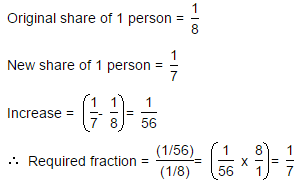QUESTION: 10

To fill a tank, 25 buckets of water is required. How many buckets of water will be required to fill the same tank if the capacity of the bucket is reduced to two-fifth of its present ?

Solution: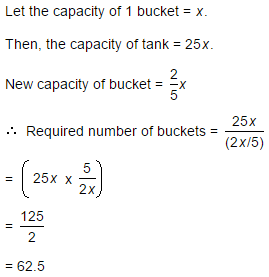Use Code STAYHOME200 and get INR 200 additional OFF Use Coupon Code